Inverse Filtering

# Inverse Filtering

If we know of or can create a good model of the blurring function that corrupted an image, the quickest and easiest way to restore that is by inverse filtering. Unfortunately, since the inverse filter is a form of high pass filer, inverse filtering responds very badly to any noise that is present in the image because noise tends to be high frequency. In this section, we explore two methods of inverse filtering - a thresholding method and an iterative method.

## Method 1: Thresholding

### Theory

We can model a blurred image by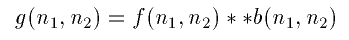where f is the original image, b is some kind of a low pass filter and g is our blurred image. So to get back the original image, we would just have to convolve our blurred function with some kind of a high pass filter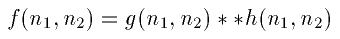But how do we find h? If we take the DFT of b so that B=DFT2(b), we would get something that looks like this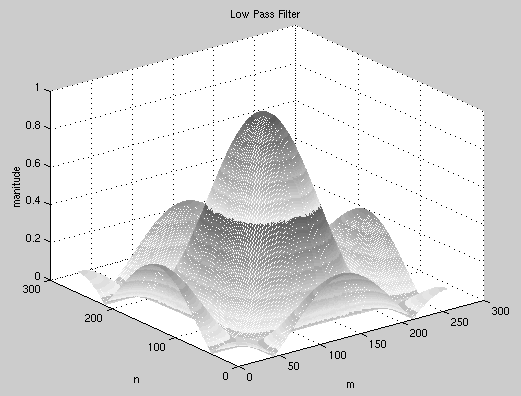In the ideal case, we would just invert all the elements of B to get a high pass filter. However, notice that a lot of the elements in B have values either at zero or very close to it. Inverting these elements would give us either infinities or some extremely high values. In order to avoid these values, we will need to set some sort of a threshold on the inverted element. So instead of making a full inverse out of B, we can an "almost" full inverse by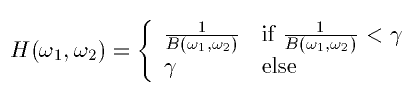So the higher we set, the closer H is to the full inverse filter.

### Implementation and Results

Since Matlab does not deal well with infinity, we had to threshold B before we took the inverse. So we did the following: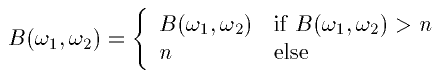where n is essentiallyand is set arbitrarily close to zero for noiseless cases. The following images shows our results for n=0.0001.We see that the image is almost exactly like the original. The MSE is 2.5847.

Because an inverse filter is a high pass filter, it does not perform well in the presence of noise. There is a definite tradeoff between de-blurring and de-noising. In the following image, the blurred image is corrupted by AWGN with variance 10. n=0.2.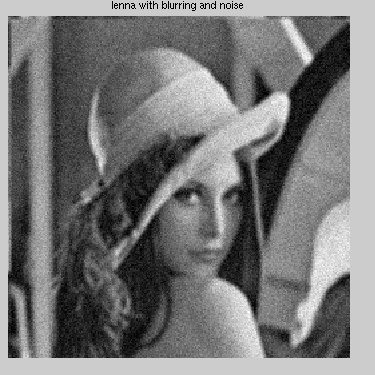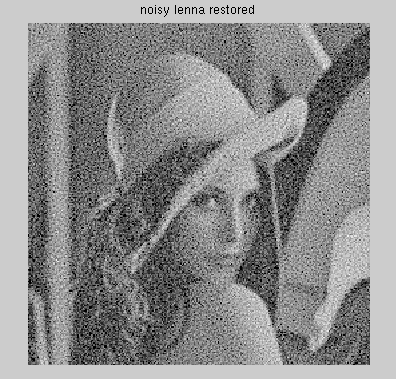The MSE for the restored image is 1964.5. We can see that the sharpness of the edges improved but we also have a lot of noise specs in the image. We can get rid of more specs (thereby getting a smoother image) by increasing n. In general, the more noise we have in the image, the higher we set n. The higher the n, the less high pass the filter is, which means that it amplifies noise less. It also means, however, that the edges will not be as sharp as they could be.

## Method 2: Iterative Procedure

### Theory

The idea behind the iterative procedure is to make some initial guess of f based on g and to update that guess after every iteration. The procedure is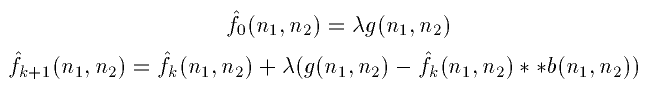where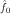is an initial guess based on g. If our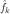is a good guess, eventuallyconvolved with b will be close to g. When that happens the second term in the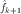equation will disappear andandwill converge.is our convergence factor and it lets us determine how fastandconverge.

If we take both of the above equations to the frequency domain, we get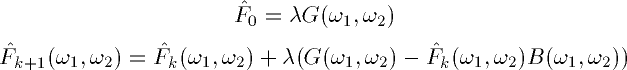Solving for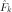recursively, we get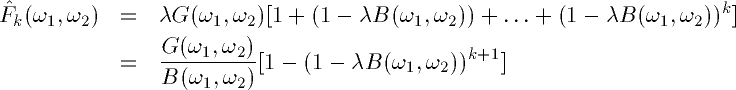So if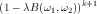goes to zero as k goes to infinity, we would get the result as obtained by the inverse filter. In general, this method will not give the exact same results as inverse filtering, but can be less sensitive to noise in some cases.

### Implementation and Results

The first thing we have to do is pick a.must satisfy the following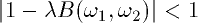and thus will be a positive integer in the range of 0 to 1. The biggeris, the fasterandwill converge. However, picking too large amay also makeanddiverge instead of converge. Imagine that we're walking along a path and the end of the path is a cliff.is the si